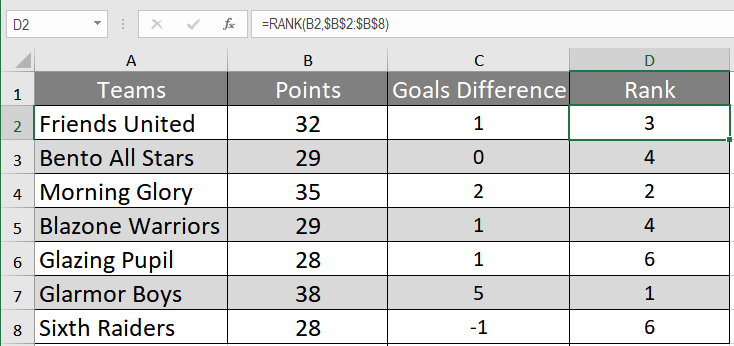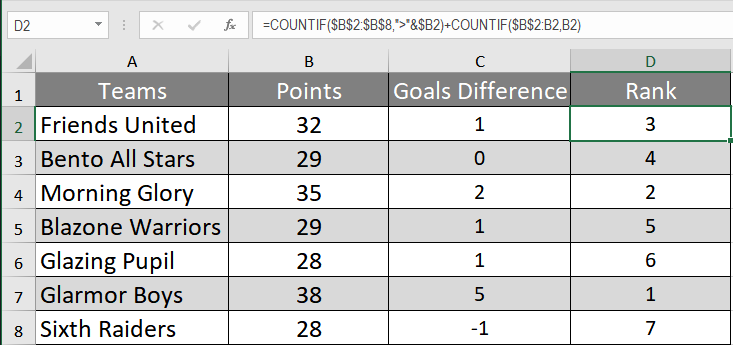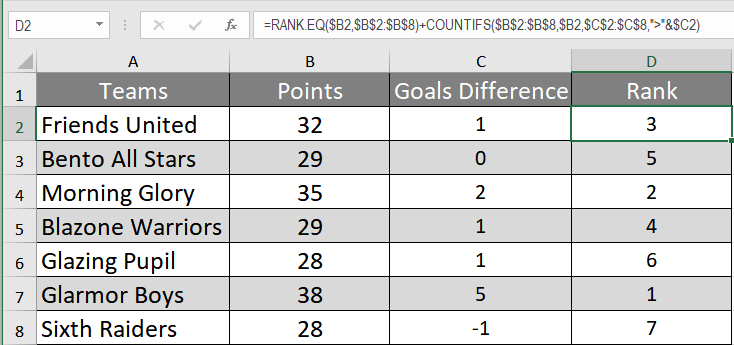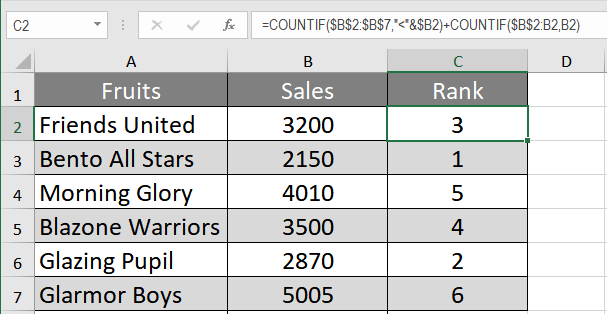Get instant live expert help with Excel or Google Sheets“My Excelchat expert helped me in less than 20 minutes, saving me what would have been 5 hours of work!”

#### Post your problem and you’ll get expert help in seconds.

Your message must be at least 40 characters
Our professional experts are available now. Your privacy is guaranteed.

# How to RANK Items in Excel Using the COUNTIFS Function

Working with data in Excel often requires you to rank data. Excel has a built-in RANK function for this purpose. But the RANK function has some limitations. One of them is RANK fails to rank data uniquely when there is duplication. You can use a neat little trick to overcome this shortcoming.

Using two COUNTIF functions together help us to rank data even if there is duplication. The COUNTIF function can also be used to rank data based on multiple criteria. In this tutorial, you will learn how to rank data using the COUNTIF function.

### How to Rank in Excel rank using the COUNTIF function

You will use a high school soccer league point table data set in the next example. There are two pairs of duplicated values in this example. Using the RANK function in column C, we get the same rank for duplicated values.To fix this issue:

• Go to cell D2 and select it with your mouse.
• Apply the formula to `=COUNTIF(\$B\$2:\$B\$8,">"&\$B2)+COUNTIF(\$B\$2:B2,B2)` cell D2.
• Press Enter.
• Drag the formula to the cells below.This will rank all the values uniquely in column C with no repetitions in descending order. The first COUNTIF function is used to find out the number of values greater or less than the number that is going to be ranked. The second COUNTIF has an expanding range \$B\$2:B2 and extracts the number of values that are equal to the number.

### Rank in Excel Using Multiple Criteria

In the previous example, Blazone Warriors are ranked lower than Bento All Stars despite having a higher goal difference. You can use the RANK.EQ with COUNTIFS to add multiple criteria to have an improved ranking using criteria. For this example:

To fix this issue:

• Go to cell D2 and select it with your mouse.
• Apply the formula `=RANK.EQ(\$B2,\$B\$2:\$B\$8)+COUNTIFS(\$B\$2:\$B\$8,\$B2,\$C\$2:\$C\$8,">"&\$C2)`to cell D2.
• Press Enter.
• Drag the formula to the cells below.This will show Blazone Warriors ahead of Bento All Stars in the rankings. The first formula with the criteria (\$B2,\$B\$2:\$B\$8) counts the number of times the values appear. The formula performs a row-wise check for the value as you have an absolute reference but a relative one for the rows (\$B2).

The second formula with the criteria (\$C\$2:\$C\$8,”>”&\$C2) checks how many goal differences are greater than the one being checked. This returns 0 for Blazone Warriors. However, Bento All Stars has 1 returned as the other team has a higher goal difference.

### Rank in an Ascending Order using the COUNTIF function

You can rank in an ascending order using the COUNTIF function. You will use the fruit sales data in this example. To rank the sales in an ascending order:

• Go to cell C2 and select it.
• Apply the formula to`=COUNTIF(\$B\$2:\$B\$7,"<"&\$B2)+COUNTIF(\$B\$2:B2,B2)` C2.
• Press Enter.
• Drag the formula with your mouse to the cells below.This will rank the sales in an ascending order.

Still need some help with Excel formatting or have other questions about Excel? Connect with a live Excel expert here for some 1 on 1 help. Your first session is always free.

### Did this post not answer your question? Get a solution from connecting with the expert.Another blog reader asked this question today on Excelchat:
Related blogs
Solution examplesI have a spreadsheet that contains a list of names/IDs and I want to generate a formula that inserts into a cell the number of times a name or ID appears on that list. Either insert a column into that spreadsheet or transfer that info into a column on a different spreadsheet. Either way would be fine.
Solved by O. L. in 18 minsHello, I have a spreadsheet with 3159 rows. One column consists of 9-digit numbers in each of the 3159 rows. I need the formula that can calculate how many of those numbers appear more than once.
Solved by D. H. in 56 minsI need a formula to count the number of rows that contain at least one of two possible phrases, but not give me the total number of instances that both phrases occur overall.
Solved by E. B. in 33 minshow can i turn my sumifs into a countifs because my sumifs works great and i also want to count the thing im summing
Solved by O. C. in 40 minsim creating a fantasy hockey model and trying to figure out how to rank if someone has played more than a certain number of games, and if they're certain position how to rank them accordingly
Solved by X. D. in 60 mins## Subscribe to Excelchat.coAnother blog reader asked this question today on Excelchat: